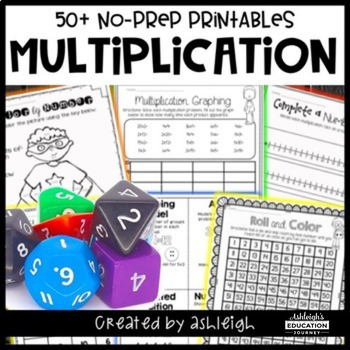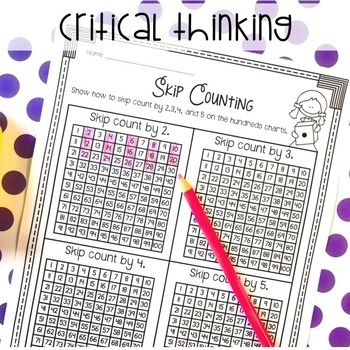# No Prep Multiplication Printables | Concept of Multiplication | Print or Digital3rd - 4th, Homeschool
Subjects
Standards
Resource Type
Formats Included
• PDF
•Google Apps™
PagesThe Teacher-Author indicated this resource includes assets from Google Workspace (e.g. docs, slides, etc.).
##### Also included in
1. FUN Math PracticeGive your students FUN math practice with these 300+ printables! This bundle contains all six of my no prep math pacts: addition and subtraction, multiplication, division, fractions, area and perimeter, and measurement.****************************************************************
\$38.60
\$49.25
Save \$10.65

### Description

Give your students FUN multiplication practice with these 50+ printables!

No Prep Needed

All you have to do is print, and you're ready to go! These multiplication printables are a great way to supplement your existing math curriculum. These worksheets were created for my students who need a little extra help with multiplication. They were designed for a third grade classroom, but can be used toward the end of the year in second grade or fourth grade students who need additional multiplication review. These worksheets are perfect for reinforcing multiplication strategies and emphasize conceptual understanding.

**************************************************************************

What's Included

Multiplication Vocabulary

Pgs. 3-5-Vocabulary Booklet

Pg. 6-Color the Array

Pg. 7-Draw the Array

Pg. 8-Read the Array

Pg. 9-Draw the Grouping Model

Pg. 10-Read the Grouping Model

Pg. 11-Complete a Number Line

Pg. 12-Read a Number Line

Pg. 13-Repeated Addition Number Sentences

Pg. 15-Representing Multiplication

Pg. 16-Understanding Multiplication

Pg. 17-Color by Number (products up to 20)

Pg. 18-Color by Number (products up to 45)

Pg. 19-Color by Number (multiples of 10)

Pg. 20-Spin and Color (products up to 20)

Pg. 21-Spin and Color (products up to 42)

Pg. 22-Spin and Color (products up to 72)

Pg. 23-Multiplication Graphing (products up to 24)

Pg. 24-Multiplication Graphing (products up to 40)

Pg. 25-Multiplication Graphing (multiples of 10)

Pgs. 26-33 (organized by factors)

Pg. 34-Roll and Color (six-sided die)

Pg. 35-Roll and Color (nine-sided die)

Pg. 36-Roll and Color (skip counting)

Pg. 37-Skip Counting

Pg. 38-Comparing Numbers-1

Pg. 39-Comparing Numbers-2

Pg. 40-Missing Numbers

Pg. 41-Input/Output Tables

Pg. 42-Cut and Paste Arrays

Pg. 43-Cut and Paste Repeated Addition

Pg. 44-Multiplication Table

Pg. 45-Multiplication Word Problems 1

Pg. 46-Multiplication Word Problems 2

Pg. 47-Products to 25

Pg. 48-Products to 81

Pg. 49-Comparing Factors

Pg. 50-Comparing Multiples

Pg. 51-Properties of Multiplication

Pg. 52-Distributive Property

Check these other math products!

Third Grade Math Assessments Test Prep Edition

Multiplication Booklets

3.OA.1

3.OA.3

3.OA.4

3.OA.5

3.OA.6

3.OA.7

3.OA.9

Total Pages
Included
Teaching Duration
N/A
Report this resource to TpT
Reported resources will be reviewed by our team. Report this resource to let us know if this resource violates TpT’s content guidelines.

### Standards

to see state-specific standards (only available in the US).
Use addition to find the total number of objects arranged in rectangular arrays with up to 5 rows and up to 5 columns; write an equation to express the total as a sum of equal addends.
Interpret products of whole numbers, e.g., interpret 5 × 7 as the total number of objects in 5 groups of 7 objects each. For example, describe a context in which a total number of objects can be expressed as 5 × 7.
Use multiplication and division within 100 to solve word problems in situations involving equal groups, arrays, and measurement quantities, e.g., by using drawings and equations with a symbol for the unknown number to represent the problem.
Determine the unknown whole number in a multiplication or division equation relating three whole numbers. For example, determine the unknown number that makes the equation true in each of the equations 8 × ? = 48, 5 = __ ÷ 3, 6 × 6 = ?.
Apply properties of operations as strategies to multiply and divide. Examples: If 6 × 4 = 24 is known, then 4 × 6 = 24 is also known. (Commutative property of multiplication.) 3 × 5 × 2 can be found by 3 × 5 = 15, then 15 × 2 = 30, or by 5 × 2 = 10, then 3 × 10 = 30. (Associative property of multiplication.) Knowing that 8 × 5 = 40 and 8 × 2 = 16, one can find 8 × 7 as 8 × (5 + 2) = (8 × 5) + (8 × 2) = 40 + 16 = 56. (Distributive property.)

### Questions & Answers

Teachers Pay Teachers is an online marketplace where teachers buy and sell original educational materials.